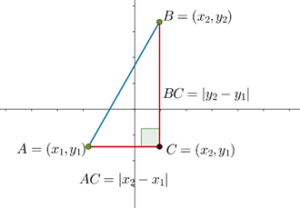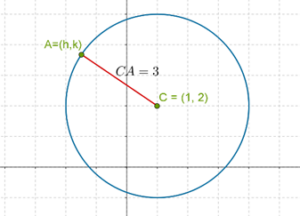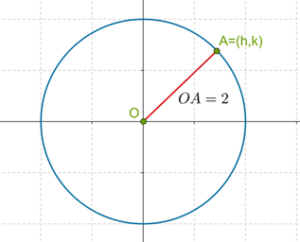In the verge of coronavirus pandemic, we are providing FREE access to our entire Online Curriculum to ensure Learning Doesn't STOP!

# The Distance Formula

Go back to  'Coordinate-Geometry'

In the previous example, we calculated the distance between two points in the plane with specific coordinates. Let us now generalize this. Suppose that we have two points with any arbitrary coordinates:

\begin{align}&A\left( {{x_1},\;{y_1}} \right)\\&B\left( {{x_2},\;{y_2}} \right)\end{align}

How do we calculate AB? Once again, we use the Pythagoras Theorem. We complete the right triangle ABC, where C is the point with the same y coordinate as A and the same x coordinate as B. Consider the following diagram:In this right triangle, note that AC is the difference between the x coordinates of A and C, and hence we write:

$AC = \left| {{x_2} - {x_1}} \right|$

Note how we have used the modulus sign, because we want the positive value of the difference between A and C. In this particular case, C is to the right of A, and so the x coordinate of C will be larger than that of A. However, in a general situation, any of the two can be larger, and so it’s a good idea to use the modulus sign.

Similarly, BC will be equal to the difference between the y coordinates of B and C. Hence, we have:

$BC = \left| {{y_2} - {y_1}} \right|$

Finally, we apply the Pythagoras Theorem to calculate AB:

\begin{align}&AB=\sqrt{AC^2+BC^2}\\&\,\;\;\;\;\;=\sqrt{\vert x_2-x_1\vert^2+\vert y_2-y_1\vert^2}\\\end{align}

\begin{array}{l}\;\Rightarrow\;\;\;\;\;\fbox{$\displaystyle AB\;\;= \sqrt{\left(x_2-x_1\right)^2+\left(y_2-y_1\right)^2\;}$} \\\end{array}

This is the widely used distance formula to determine the distance between any two points in the coordinate plane. Note that in the final expression, we removed the modulus signs, since the terms got squared – so it doesn’t matter whether the original terms are negative or positive. For example, the square of 2 is 4, the same as the square of –2.

Example 1: Find the distances between the following pairs of points:

\begin{align}&({\rm{i}})\,\,A\left( {2,\;4} \right),\;B\left( {3,\;2} \right)\\&({\rm{ii}})\,\,C\left( { - 1,\; - 3} \right),\;D\left( {0,\;5} \right)\\&({\rm{iii}})\,E\left( {0,\;\sqrt 2 } \right),\;F\left( { - 1,\;3\sqrt 2 } \right)\\&({\rm{iv}})\;G\left( {0,\;0} \right),\;H\left( { - \frac{1}{2},\;\frac{{\sqrt 3 }}{2}} \right)\end{align}

Solution: In each case, we apply the distance formula:

\begin{align}&({\rm{i}})\,\,AB = \sqrt {{{\left( {2 - 3} \right)}^2} + {{\left( {4 - 2} \right)}^2}} = \sqrt {{1^2} + {2^2}} = \sqrt 5 \\&({\rm{ii}})\,\,CD = \sqrt {{{\left( { - 1 - 0} \right)}^2} + {{\left( { - 3 - 5} \right)}^2}} = \sqrt {{1^2} + {8^2}} = \sqrt {65} \\&({\rm{iii}})\,\,EF = \sqrt {{{\left( {0 - \left( { - 1} \right)} \right)}^2} + {{\left( {\sqrt 2 - 3\sqrt 2 } \right)}^2}} \;\; = \sqrt {{1^2} + {{\left( {2\sqrt 2 } \right)}^2}} \\& \,\;\;\;\;\qquad\qquad\qquad\qquad\qquad\qquad\qquad\qquad\quad = \sqrt {1 + 8} \; = 3\\&({\rm{iv}})\,GH = \sqrt {{{\left( {0 - \left( { - \frac{1}{2}} \right)} \right)}^2} + {{\left( {0 - \frac{{\sqrt 3 }}{2}} \right)}^2}} \; = \sqrt {{{\left( {\frac{1}{2}} \right)}^2} + {{\left( {\frac{{\sqrt 3 }}{2}} \right)}^2}} \\ &\;\;\;\;\;\,\qquad\qquad\qquad\qquad\qquad\qquad\qquad\qquad\qquad = \sqrt {\frac{1}{4} + \frac{3}{4}} \; = 1\end{align}

Example 2: A triangle has the following vertices:

$$A\left( {0,\;1} \right),\;B\left( {2,\;3} \right),\;C\left( {4,\; - 1} \right)$$

What is the perimeter of this triangle?

Solution: We calculate the length of each side using the distance formula:

\begin{align}&AB\;\;=\sqrt{\left(0-2\right)^2+\left(1-3\right)^2}\\&\qquad=\sqrt{2^2+2^2}\;=\sqrt8\;=2\sqrt2\\&BC\;\;=\sqrt{\left(2-4\right)^2+\left(3-\left(-1\right)\right)^2}\\&\qquad=\sqrt{2^2+4^2}\;=\sqrt{20}\;=2\sqrt5\\&AC=\sqrt{\left(0-4\right)^2+\left(1-\left(-1\right)\right)^2}\\&\qquad=\sqrt{4^2+2^2}\;=\sqrt{20}\;=2\sqrt5\end{align}

Thus, the perimeter is

\begin{align}&P = AB + BC + AC\\&\quad = 2\sqrt 2 + 2\sqrt 5 + 2\sqrt 5 \\&\quad= 2\left( {\sqrt 2 + 2\sqrt 5 } \right)\;{\rm{units}}\end{align}

Example 3: Consider a point A with the coordinates $$\left( {h,\;k} \right)$$, where h and k can vary. However, h and k always satisfy the following constraint:

$${h^2} + {k^2} = 4$$

What can be the possible locations of point A in the plane?

Solution: We write the given constraint as follows:

\begin{align}&{\left( {h - 0} \right)^2} + {\left( {k - 0} \right)^2} = 4\\ &\Rightarrow {\left( {h - 0} \right)^2} + {\left( {k - 0} \right)^2} = {2^2}\\ &\Rightarrow \sqrt {{{\left( {h - 0} \right)}^2} + {{\left( {k - 0} \right)}^2}} = 2\end{align}

Now, look at the expression on the left side carefully, and compare it with the expression of the distance formula. It is easy to figure out that the left side expresses the distance between the point $$\left( {h,\;k} \right)$$ and the origin, that is, $$\left( {0,\;0} \right)$$. So, what the equality above says is that the distance of $$\left( {h,\;k} \right)$$ from $$\left( {0,\;0} \right)$$ is fixed, and equal to 2. This means that the location of A should be such that its distance from the origin must be 2 units. Thus, A can lie anywhere on a circle of radius 2 units centered at the origin:Example 4: Bono is walking in the plane, such that his coordinates $$\left( {h,\;k} \right)$$ always satisfy the following constraint:

$$\sqrt {{{\left( {h - 1} \right)}^2} + {{\left( {k - 2} \right)}^2}} = 3$$

What is Bonos path in the plane?

Solution: Once again, compare the expression on the left side in the given equality to the distance formula. It is easy to see that this represents the distance between the point $$\left( {h,\;k} \right)$$ and the point C with the coordinates $$\left( {1,\;2} \right)$$. The given equality thus says that the distance between $$\left( {h,\;k} \right)$$ and $$\left( {1,\;2} \right)$$ is fixed, and is equal to 3. This means that howsoever Bono moves in the plane, the distance of his position A from the point $$\left( {1,\;2} \right)$$ must be fixed at 3 units. Thus, Bono must move on a circle of radius 3 units centered at the point C, that is, $$\left( {1,\;2} \right)$$:Coordinate Geometry
Grade 9 | Questions Set 1
Coordinate Geometry
Coordinate Geometry
Grade 10 | Questions Set 1
Coordinate Geometry